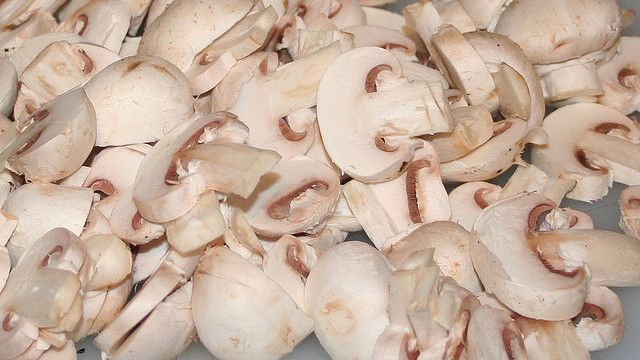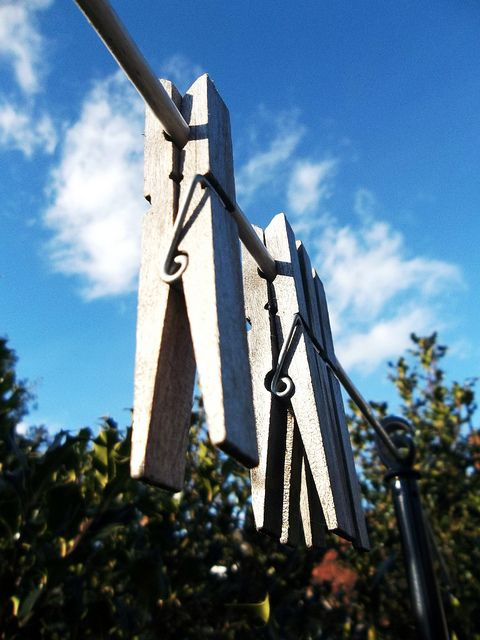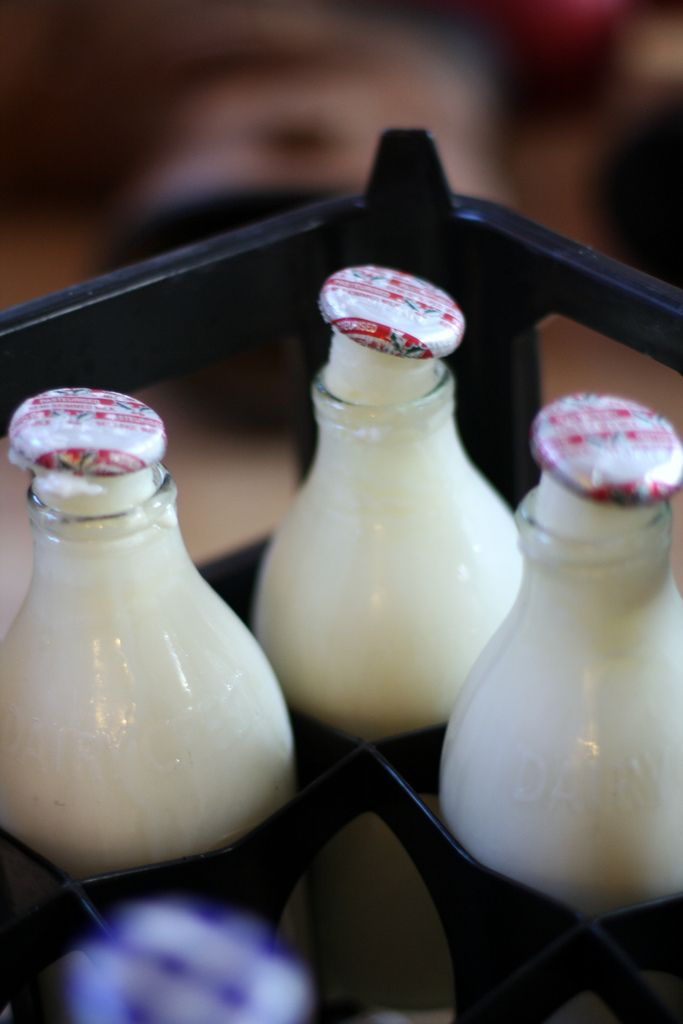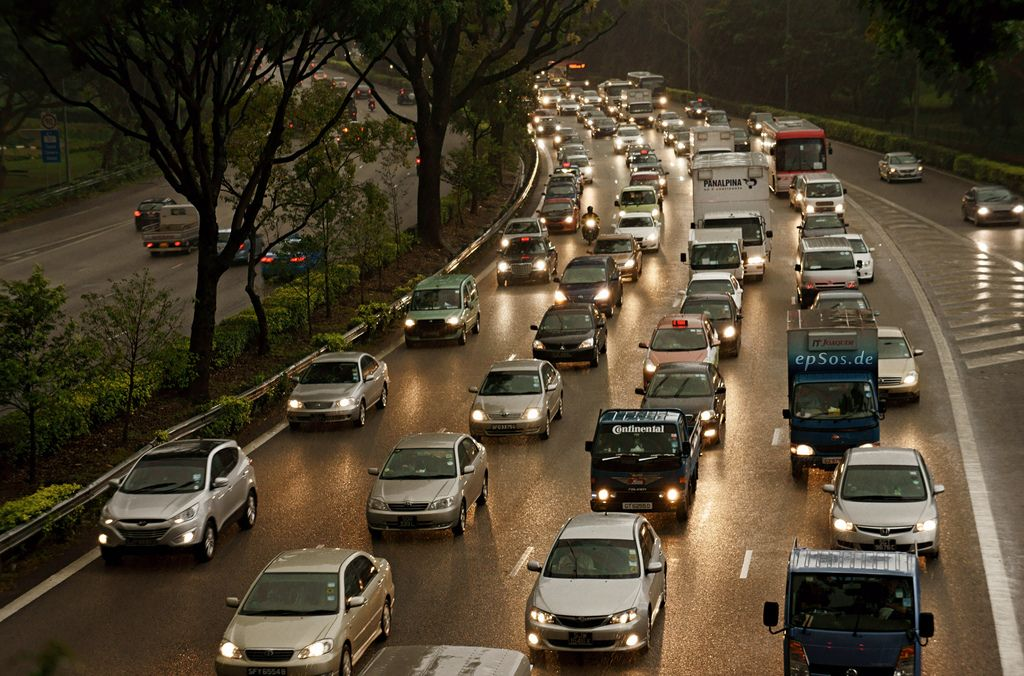Home Practice
For learners and parents For teachers and schools
Textbooks
Full catalogue
Pricing SupportLog in

We think you are located in United States. Is this correct?

# End of chapter activity

Exercise 1.12

Write the following numbers in order from biggest to smallest:

$$\text{365 280}$$; $$\text{635 765}$$; $$\text{6 650 232}$$; $$\text{3 695 212}$$; $$\text{5 355 005}$$

$$\text{6 650 232}$$; $$\text{5 355 005}$$; $$\text{3 695 212}$$; $$\text{635 765}$$; $$\text{365 280}$$;

$$\text{27,28}$$; $$\text{1 278}$$; $$\text{872}$$; $$\text{78,2}$$; $$\text{7 812}$$; $$\text{28,27}$$

$$\text{7 812}$$; $$\text{1 278}$$; $$\text{872}$$; $$\text{78,2}$$; $$\text{28,27}$$; $$\text{27,28}$$;

$$\text{8 903}$$; $$\text{893}$$; $$\text{89,30}$$; $$\text{89,89}$$; $$\text{9 988}$$; $$\text{3 989}$$

$$\text{9 988}$$; $$\text{8 903}$$; $$\text{3 989}$$; $$\text{893}$$; $$\text{89,89}$$; $$\text{89,30}$$

$$\text{12 345}$$; $$\text{120 345}$$; $$\text{120,54}$$; $$\text{542 120}$$; $$\text{55 420}$$

$$\text{542 120}$$; $$\text{120 345}$$; $$\text{55 420}$$; $$\text{12 345}$$; $$\text{120,54}$$

Rewrite the following calculations with brackets to make the answers correct:

$$\text{23} + \text{6} \times \text{5} = \text{145}$$

$$(\text{23} + \text{6}) \times \text{5} = \text{145}$$

$$\text{12} + \text{2} \times \text{82} = \text{176}$$

$$\text{12} + (\text{2} \times \text{82}) = \text{176}$$ OR $$\text{12} + \text{2} \times \text{82} = \text{176}$$ (brackets not needed)

$$\text{18} + \text{3} \times \text{17} = \text{69}$$

$$\text{18} + (\text{3} \times \text{17}) = \text{69}$$ OR $$\text{18} + \text{3} \times \text{17} = \text{69}$$ (brackets not needed)

$$\text{18} + \text{3} \times \text{17} = \text{357}$$

$$(\text{18} + \text{3}) \times \text{17} = \text{357}$$

$$\text{15} + \text{7} \times \text{5} = \text{110}$$

$$(\text{15} + \text{7}) \times \text{5} = \text{110}$$

$$\text{65} \times \text{2} + \text{5} = \text{455}$$

$$\text{65} \times (\text{2} + \text{5}) = \text{45}$$$$\text{5}$$

$$\text{115} + \text{4} \times \text{12} = \text{163}$$

$$\text{115} + (\text{4} \times \text{12}) = \text{163}$$ OR $$\text{115} + \text{4} \times \text{12} = \text{163}$$ (brackets not needed)

For each of the following questions, calculate the answer and then round it up or down depending on the situation. Explain why you rounded the way you did.

Liam is packing mushrooms. There are $$\text{15}$$ mushrooms in a punnet. He has $$\text{275}$$ mushrooms to pack. How many punnets does he need?$$\text{275} \div \text{15} = \text{18,33}\ldots$$ He can only sell full punnets, so he must round down to $$\text{18}$$.

A car mechanic charges $$\text{R}\,\text{200}$$ per hour or part thereof for labour. How much would he charge for a job that takes $$\text{270}$$ minutes?

$$\text{270}$$ minutes is $$\text{4}$$ hours and $$\text{30}$$ minutes, but he rounds up to $$\text{5}$$ hours, as he charges “per hour or part thereof”. So he charges $$\text{R}\,\text{1 000}$$.

An amusement park ride has places for $$\text{30}$$ people. $$\text{82}$$ people are in the queue for the ride. How many times will the ride need to run?

$$\text{82} \div \text{30} = \text{2}$$ remainder $$\text{22}$$. They cannot turn away customers, so they should round up and run the ride $$\text{3}$$ times.

Nokuthula is putting up washing lines in her yard. The distance from one pole to the other is $$\text{3,2}$$ $$\text{m}$$. How many lines can she put up if she has $$\text{18}$$ $$\text{m}$$ of washing line?$$\text{18} \div \text{3,2} = \text{5,625}$$. She should round down, as she can't put up a fraction of a line, and may need more line for knots and so on. So she can hang $$\text{5}$$ lengths of line.

How much would a customer pay for each of these totals in a shop?

$$\text{R}\,\text{215,67}$$

$$\text{R}\,\text{215,65}$$

$$\text{R}\,\text{329,29}$$

$$\text{R}\,\text{329,25}$$

$$\text{R}\,\text{65,33}$$

$$\text{R}\,\text{65,30}$$

For each of the following problems, first write two ratios equal to each other, then find the unknown value:

A recipe calls for $$\frac{\text{1}}{\text{3}}$$ cup sugar to $$\text{2}$$ cups of flour. How many cups of flour do you need to add to $$\text{3}$$ cups of sugar?

$$\frac{\text{1}}{\text{3}} : \text{2} = \text{1} : \text{6} = \text{3} : \text{18}$$. So you need to add $$\text{18}$$ cups of flour to $$\text{3}$$ cups of sugar.

A survey shows that $$\text{5}$$ : $$\text{1}$$ learners in a school have their own cell phone. If there are $$\text{1 350}$$ learners in the school, how many do not have their own cell phone?

There are $$\text{6}$$ parts in the ratio, so $$\text{1}$$ part $$= \text{1 350} \div \text{6} = \text{225}$$ learners do not have their own cell phone.

Three litres of milk cost $$\text{R}\,\text{29,95}$$ at Shop A and two litres of milk cost $$\text{R}\,\text{15,95}$$ at Shop B.

What is the cost per litre at each shop?

Shop: A $$\text{R}\,\text{29,95} \div \text{3} = \text{R}\,\text{9,98}$$. Shop B: $$\text{R}\,\text{15,95} \div \text{2} = \text{R}\,\text{7,98}$$

Shop B.

How much would five litres of milk cost at each shop?Shop A: $$\text{R}\,\text{9,98} \times \text{5} = \text{R}\,\text{49,90}$$. Shop B: $$\text{R}\,\text{7,98} \times \text{5} = \text{R}\,\text{39,90}$$

Two different sized jars of jam are sold at the following prices: A: $$\text{500}$$ $$\text{g}$$ for $$\text{R}\,\text{8,50}$$ or B: $$\text{750}$$ $$\text{g}$$ for $$\text{R}\,\text{11,50}$$. Which size is the better buy?

Work out what $$\text{100}$$ $$\text{g}$$ costs for each. A: $$\text{R}\,\text{8,50} \div \text{5} = \text{R}\,\text{1,70}$$ and B: $$\text{R}\,\text{11,50} \div \text{7,5} = \text{R}\,\text{1,533} \ldots$$ So jar B is cheaper.

Do these calculations without a calculator:

$$\text{240,01} \times \text{100}$$

$$\text{24 001}$$

$$\text{364,5} \times \text{1 000}$$

$$\text{364 500}$$

$$\text{1 865,03} \times \text{10}$$

$$\text{18 650,3}$$

$$\text{990,13} \times \text{1 000}$$

$$\text{990 130}$$

$$\text{5,298} \times \text{100}$$

$$\text{529,8}$$

$$\text{6 995,86} \div \text{1 000}$$

$$\text{6,99586}$$

$$\text{3 784,41} \div \text{100}$$

$$\text{37,8441}$$

$$\text{788,1} \div \text{1 000}$$

$$\text{0,7881}$$

The following numbers are not perfect squares. Calculate the square roots of these numbers (using a calculator) and give the answer rounded off to two decimal places if necessary.

$$\text{222}$$

$$\text{14,90}$$

$$\text{845}$$

$$\text{29,07}$$

$$\text{6 120}$$

$$\text{78,23}$$

$$\text{44 032}$$

$$\text{209,84}$$

The price of a new car is $$\text{R}\,\text{210 000}$$. Mr Simelane is offered a $$\text{12}\%$$ discount. How much will he pay?

$$\text{R}\,\text{210 000} - \text{12}\% = \text{R}\,\text{184 800}$$

A packet of rice weighs $$\text{1,5}$$ $$\text{kg}$$ when it is bought. Some of the rice has been used and the packet now weighs $$\text{15}\%$$ less. What is the weight of the rice that was used?

$$\text{1,5}\text{ kg} - \text{15}\% = \text{1,275}\text{ kg}$$. $$\text{1,5}\text{ kg} - \text{1,275}\text{ kg} = \text{0,225}\text{ kg}$$ of rice was used.

The price of a TV set is $$\text{R}\,\text{2 786}$$. If a buyer is offered an $$\text{11}\%$$ discount, what does he pay for it?

$$\text{R}\,\text{2 786} - \text{11}\% = \text{R}\,\text{2 479,54}$$

A car was valued at $$\text{R}\,\text{175 000}$$ when it was purchased. After three years it was sold for $$\text{R}\,\text{82 000}$$. What percentage of its original value did the car lose?$$\text{R}\,\text{175 000} - \text{R}\,\text{82 000} = \text{R}\,\text{93 000}$$. $$\frac{\text{93 000}}{\text{175 000}}\% = \text{53,14}\%$$